# Shaolin vs Wutang 2: Full Combos (All Characters)

You ask for it, you got it! A full Combo Guild for all characters! Bread and butter combos. Hard to figure out combos. Hidden moves. overheads. Lows. Unblockable moves and more.

### Explanation of Terms and the guild

P Punch
K Kick
W Weapon
F forward
B back
D down
U up
QCB= quarter circle back
QCF=quarter circle forward
* after a down direction input means you hit the next button while rising
) after an direction input means to hold in that direct for the whole combo string
+ means at the same time
> Means it’s one of the things it combos into
The first button or combonation in a sentence is what will combo into everthing starting with an > in that line.
~ means it appears to combo into it( atleast with button mashing)but doesn’t register as a combo so it can be blocked by your opponant.
OR- another option for the last button in a combo or after the last coma
>/~ sometimes it will combo for me and other times it will not or has a very strink timing.
Overhead- needs to be blocked high
Low- needs to be blocked low
I tend to use fast button mashing to get combo to come out (I’ll hit more buttons than nessesary than switch to the next button based on what I see on the screen)but if you don’t do that you’ll need to have good timing for buttons to combo. Hit the button at the end of the animation of the last button pushed.
I’m not going to list everything in the move list in the game so check there for the basics. I list the specials just because curently not every character has a special for all the possible imputes and some combo into other things. I list when that is the case.
I usually only include the longest imput string possible unlest it results in and origional move or it has only certain or unique things it can combo into. but a lot of times you can use shorter strings to start out the combo or in the middle of a combo that is long or you can do into a combo ender at any time where it will connect.
I want this guild to get more player into this game and to be amazed by it like I am. If there was an online mode for this game or if there is one in the future I might regret this guilde because these are combos I’ve figure out that would have given me an edge online. But I would rather the comunity grow and benifit from this guilde.Not everone has the time to sit down and figure out combos and it can be easy to forget them when you do find a great one or you think to yourself how did I do that? So this will be a referance for times.If you want me to add a combo to this guild leave a comment for me. I might have not included a combo if it can be done by one of the combo enders I’ve listed. The goal with this guild was to show you combos that you might have not thought of. And to give you ideas not to be a complete combo list for each character. The combo system is to open to be able to list ever single combo but Ive done my best to list the best ones. Enjoy!
Notes: Throws don’t appear to work on crouching opponents. Couching Low Punches appear to need to be blocked low. Jumping normals don’t appear to be over heads but jumping weapon attacks are.
Sugestions: I think it would be cool if characters who’s Dash,Dash goes airborne that they could use an attack but atleast it go over sweeps.

### American Karate

American Karate
D*W (LOW)
W >U+W <D+W
WW >U+W >D+W ~B+W
WWW >/~D+W ~W(OVERHEAD) ~U+P ~B+P >F)+WWW or U+W or ~W
F+W >U+W ~B+W
QCB+P >QCF+P
QCF+P
D*P
P >D+P >B+P
PP >+P >D+P >B+P >U+P(OVERHEAD) F)+KKKK >KKKK >F)+KK or B+K OR U+K OR B+K >D+K,KK or BK >F)+PPP
PPP >P(OVERHEAD) >B+P >U+P >F)+PPP ~W >F)+PPP,F)+KKKK
F)+P >PP
>F+KK,D+K,KK or B+K or B+P OR D+P
K >PP >U+K >B+K >B+P >U+P(OVERHEAD) ~B+W >D)+KK,B+K or >/~K
KK >PP >U+K >B+K >B+P >U+P ~B+W >D)+KK,B+K or >/~K
KKK ~K(OVERHEAD) >D)+KK,B+K or >/~K >
D*K(LOW)>K,K(OVERHEAD) >B+P >U+P ~B+W >KK >B)+KK
F+K >B+K OR U+K OR B+K >D+K,KK or BK >PP >F)+PPP
F)+KK >KK >F)+KK or B+K OR U+K OR B+K >D+K,KK or BK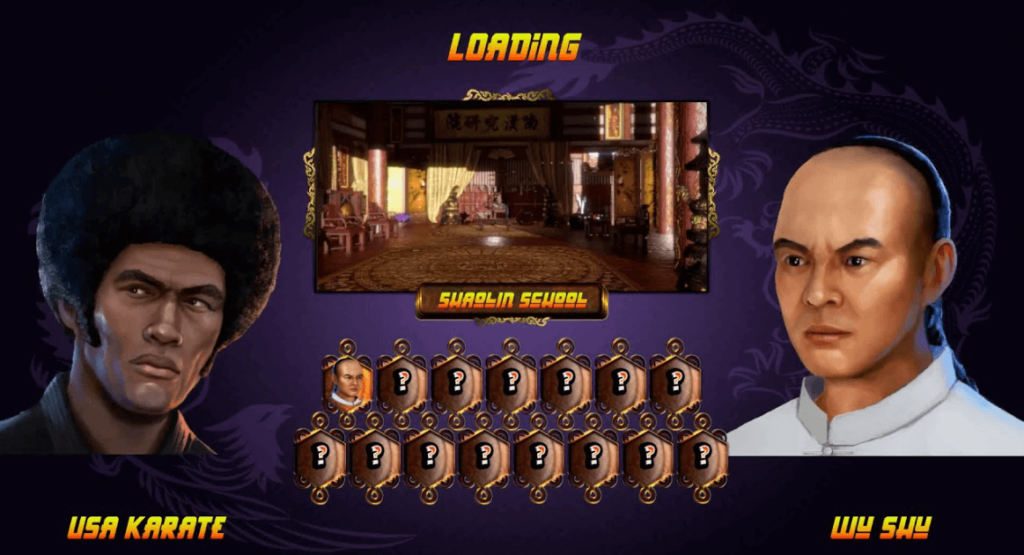### Kyokushin Karate

Kyokushin Karate
D*W (LOW)
WWWW >W >F)+WW >U+W >B+W >F+W
QCB+P(OVERHEAD) (At the very end of the animation)>QCF+P
QCF+P
P >P >U+P >D)+PP >B+W
PP ~P >D)+PP >D+P,U)+KK >B+W -F)+PP >F+P >U+P >B+P >B+W
PPP ~P(OVERHEAD) >U+P >B+P >D)+PP >B)KK >U)+KK >F)+KKKK >B+W -F)+PP >F+P >U+P >B+P
>B+W
D*P, >D+P; >B+P > U+P >U+K U+K
F+P >P(OVERHEAD) >U+P >B+P >B+W >KK~K
QCB+K
QCF+K
KK >U+K >F)+KK,D+K,B+KK >B+W ~P
KKK >K >B)+KK ~U)+KK ~P -D+K ~K >B)+KK >B+K,U+K ~U+K
F+K >KK~K >U)+KK >D+K >D+K,B)+KK
F+KK >U)+KK >D+K >D+K,B)+KK
F)+KK(OVERHEAD)K >F+K(might not combo in gaurd) >D+K,B)+KK D+K,U+K >U)+KK >B)+KK
D*K(LOW) ~K >B)+KK >B+K,U+K(doesn’t combo on gaurd) or B+P ~U+K
Notes: It looks like his super hits low but it does not.I wish he had 2 dash attacks. His current one changed to after DASH,DASH and another one done by Dash and attack. I wish He had his follow up >P for QCB+P like in Fighters Legacy.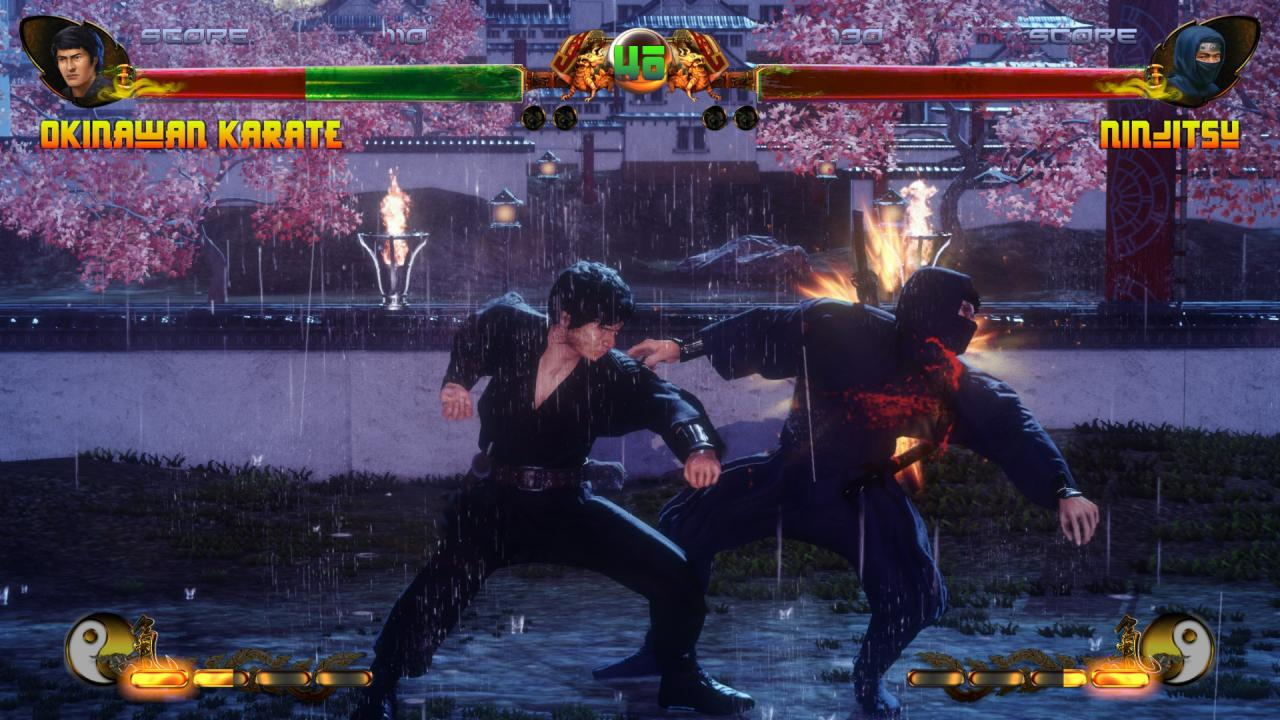### Kickboxing

Kickboxing
WW >W(Misses on crouch) >U+W >D+W >B+W B+P >F)+WWW OR >U+W >D+W >B+W
F)+WW >F+W(OVERHEAD) >U+W >D+W >B+W >B+P >B)+KK OR D+K,K
D*W (LOW)
QCF+P
D*P(LOW) >D+K
P >U)+PP OR K OR B+K >D+P,D+K >D+K,K OR D+K
PP >F+PP,F)+KK_K OR D+K,K OR U)+KK
PPP >B+P >D+P,D+K >U)+PP OR K
F)+PP >P >U+P >B+P >BK
QCB+K
QCF+K
K >P,P(OVERHEAD)or K or U)+KK or B+W >B+P >U)+PP OR K >D)+PK >B+W >D+W >U+W
KK see KKKK
KKK see KKKK
KK(LOW)KK >K >P >B+W >D+W >U+W >F)+KKK OR D)+KK >U)+KK OR K
F+K >P >K >F+KK OR D+K,K >U)+PP
B+K >K(OVERHEAD) >B+P >U+W >D+W >B+W >D)+KK OR standing K
D*K(NOT LOW) >K >B+P
Notes: Counter goes over crouched opponents.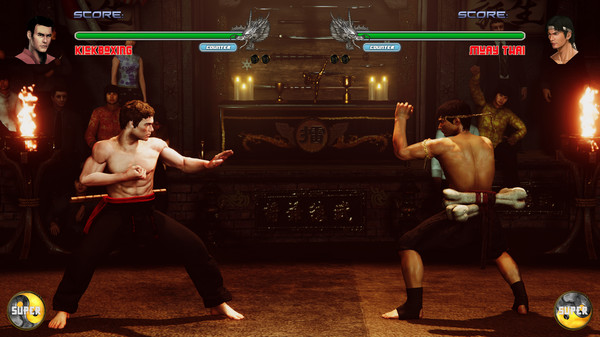### Jeet Kune Do

Jeet Kune Do
F)+WW >W >B+W >D+W ~U+W >K,F+K >K,U)+KK >K,U+K,k
WW >B+W ~U+W >F+WWW >K,F+K >K,U)+KK >K,U+K,k
WWW ~W >F+WWW ~B+W ~U+W >K,F+K >K,U)+KK >K,U+K,K
QCB+P >P >QCF+P
QCF+P
P >KPP >F)+KK >B+W
PP >U+P ~B+W
PPP >D)+PP >F+PPPPP
F+P >B+W ~B+P >K >B+K ~F+K ~PP >U)+KK
F)+PP >B+W >B+P >K >B+K >F+K >U)+KK >U+K,K,F+K
F)+PPP >K >B+K ~F+K >U)+KK >U+K,K,F+K
F)+PPPP >K >B+K ~F+K >U)+KK >U+K,K,F+K
F)+PPPPP >P(OVERHEAD) >K >F+K >B+P >B+K >U+K >U)+KK >U+K,K,F+K >U+K,F+P >B+W
PPPP >P >B+W
D*P(NOT LOW) >P >U+P >U+W >KK >F+K(LOW)>U)+KK >B+W
QCB+K(is not unblockable like a throw)
D*K >D)+KKKK ( last hit is OVERHEAD)
K >U+K >U)+KK U+K,K,F+K >B+W >PP(OVERHEAD)
KK >B+W >PPP >PP,B+P >B+W >U+P D)+KK(OVERHEAD)
KKK >K >B+K >U+P
U+K >U+K >K,K >K,F+K >F+P >D+P ~D+K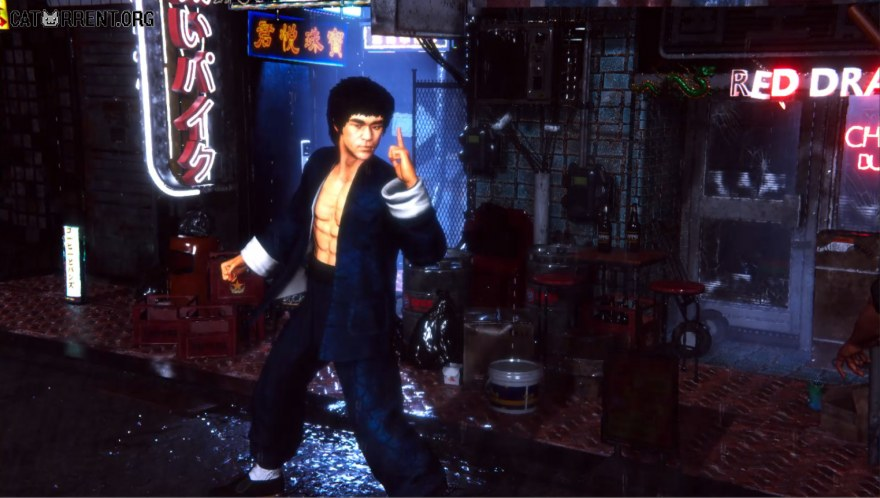### Shorinji Kempo

Shorinji Kempo
QCB+W
QCF+W
D*W (LOW)
U+W,D+W(LOW)
WW >W >U+W,D+W >F)+WW OR U+W,D+W OR B+W >F+W,U+W,D+W
B+W alternate combo ender for the last hit of a kick or punch combo
QCB+P
QCF+P
D*P
PPP >P >F)+PPP >U)+PPPP >B)+PP >D+P >KKKK >F)+KKKK >B)+KK >D)+KK >U)+KKK
F)+PP ~K
F)+PPP >F+P >PPP,B+P or D+P or B)+KK or B+W >F)+KKKK >B)+KK >D)+KK >U)+KKK >B+W
B+P >B+P >B+K >B+W
D+P COMBO alternate combo ender for the last hit of a kick or punch combo
KKK >K >B)+KK >D)+KK >U)+KKK >F)+KKKK OR D)+KK OR B)+KK OR U)+KK >D+P >B+PP >U+PPPP
D)+KKK >K(OVERHEAD)>F+P,P,P,D+P OR B)+PP OR B)+KK OR B+W >PPP,B+P or D+P or B)+KK or B+W
B)+KK
F)+KKK >K >B)+KK D)+KK U)+KKK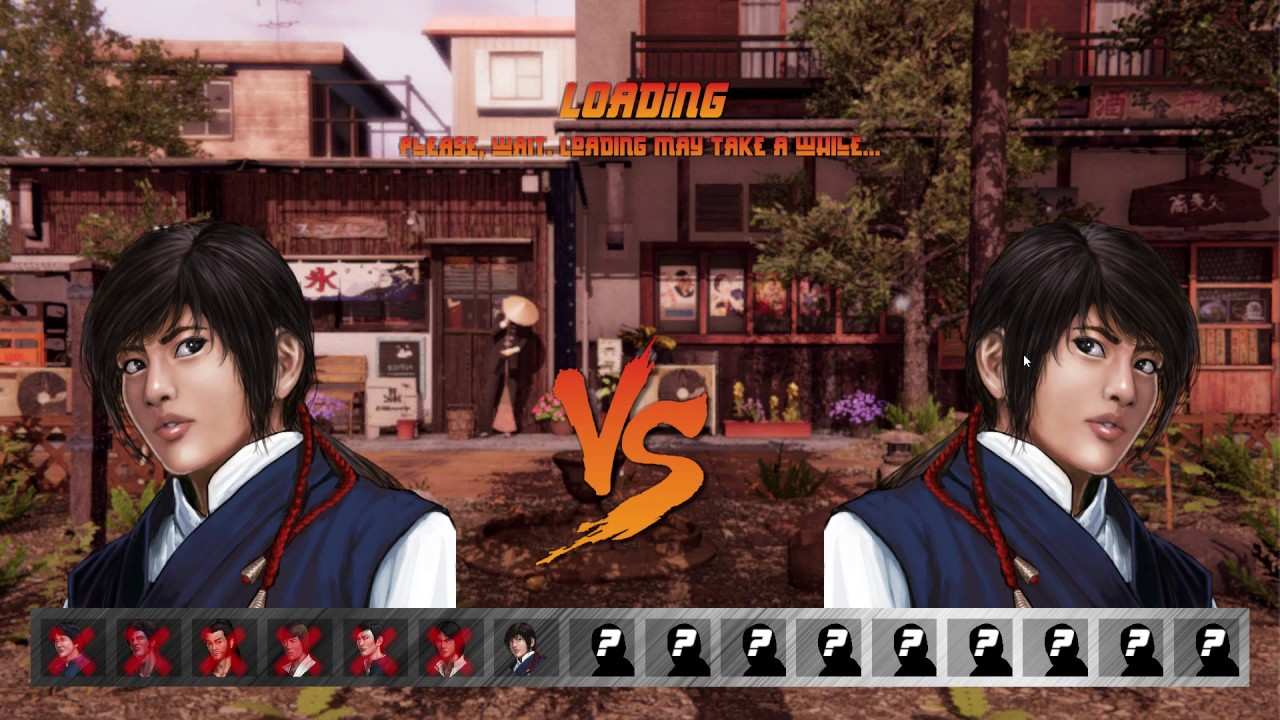### Leopard Style

Leopard Style
DASH >K (APEARS TO BE UNBLOCKABLE) *Maybe needs mixed
U+W ( goes over crouchers)
W >F+W >U+W >B+W >D+W
D*W (LOW) (APPEARS TO BE UNBLOCKABLE) *Maybe needs fixed
F+W alternate combo ender for the last hit of a kick or punch combo
QCF+P (NOT LOW)
D*P(LOW) >P alternate combo ender for the last hit of a kick or punch combo
PPPP >B)+PPP U)+PPPP
PPPPP >P >F)+PPPPP F+PPP~PP
F)+PPPP >P >F)+KKK >B)+KK
F+PPP~PP
F)+P >PP >K >PP,U)+PPPP
D+P >P >D+K alternate combo ender for the last hit of a kick or punch combo
QCB+K
QCF+K
D*K (LOW)
B)+KK
U+K ~K~K
U)+KK ~K,B+KK
KK >B)+PPP
KKK >K >F)+KKK >B+KKK >U)+KK >D+K >PP >U)+PPPP
F)+K >K,P OR D+K OR ~K >K,U+KK >B)+PPP >/~U)+PPPP >K,B)+KK
F)+KK >K(LOW) ~B+KK
D+K alternate combo ender for the last hit of a kick or punch combo
Note: Super appears to hit low but doesn’t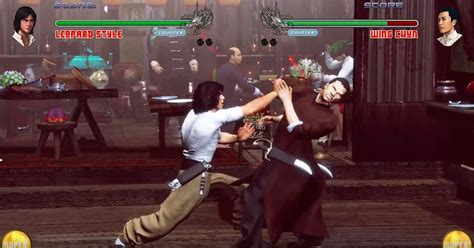### Wing Chun

Wing Chun
D*W
B+W alternate combo ender for the last hit of a kick or punch combo
QCB+P
PPPP >F)+PPPP >U)+PPPP >D+P >U+K >B+K >D)+KK >F)+KKK >KK,B)+PP or another combo ender
U+P,K
U)+PP >B)+PP >B+P >B)+W
B+P >P >B+K >U+K >B+W
D*P(LOW) B)+PP F)+PPPP >B)+W >B+K >F)+KKK
D+P alternate combo ender for the last hit of a punch combo only
B+P alternate combo ender for the last hit of a kick or punch combo
QCF+K
K >B+K >U+K >D)+KK >U)+PPPP B+W
KK(2ND HIT LOW) >K >U+K >B+K >D+K,K or B+W >B)+PP >B+W >F)+KKK or U+K or B+K >)+PPPPPP
B+K or U+P or D)+KK or K)+KKK alternate combo ender for the last hit of a kick or punch combo
D*K(LOW) >K >B+K >U+K >B)+W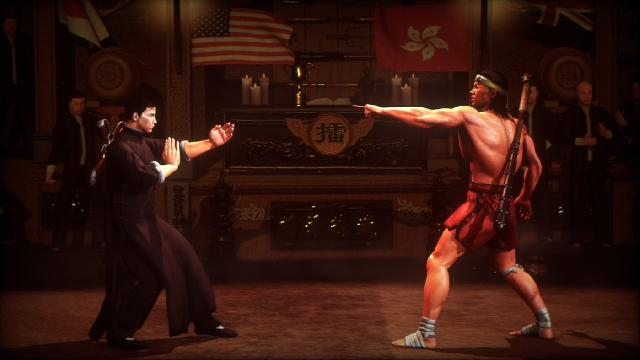### Lotus Palm

Lotus Palm
QCF+W
QCB+W
D*P >D)+PPP OR B)+PPP
W >D+W >B)+WW
WW >W >B)+WW
QCF+P
PPPP
PP U)+PP >F)+PPPP >B)+PPP D)+PPPP >U+K B)+KKK
PPP U)+PP D)+PPPP >U+K B)+KKK ~PPP
U+P >P >K >B)+WW alternate combo ender for the last hit of a kick or punch combo
B)+PP >P >B)+KKK
D*P D)+PP,P or B)+PPP >B)+PPP >B+WWW
D)+PPPP alternate combo ender for the last hit of a kick or punch combo
QCB+K
QCF+K >K
KKK ~K >U+K >B)+KKK ~D)+KKKK
B+KKK
F+K >K
F)+KK >U+K
F)+KKK >K ~U+K ~D+K ~
D*K >P >B)+WW B)+KKK U)+KKK B)+PP B)+WWW ~KKK
D+K >P >B)+WW >KKK alternate combo ender for the last hit of a kick or punch combo
D)+KKKK
notes:
If you try to combo any punches or kicks back to her neutral punches she does 3 punches starting with a spinning back first and it never combos or has very strinked timing. This is the only thing I can get to combo. F)+PP >PPP
A lot of her stuff goes into other stuff but doesn’t actually register as a real combo with the combo counter. I think I found everthing that can combo but it would be easy to miss something with her.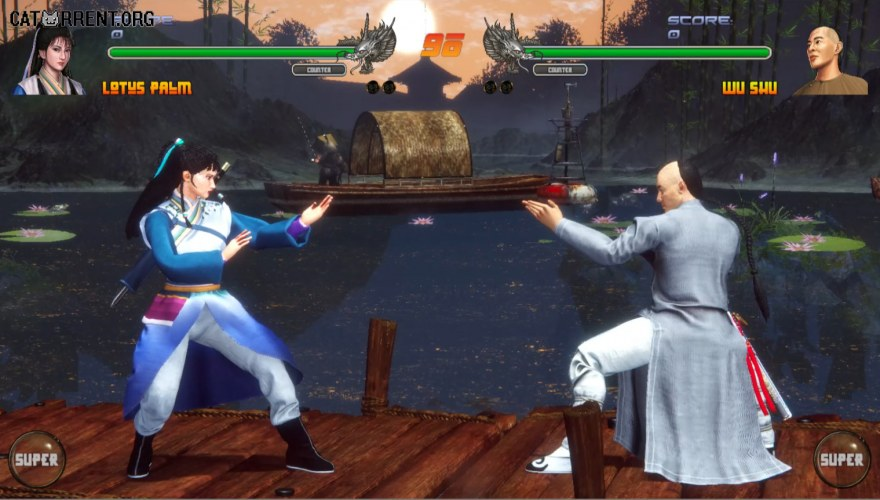### Wrestling

Wrestling
QCF+W
W >W >U+W >D+W ~B+W
F)+W >W >U+W >D+W >B+W
D*+W (LOW)
D+W >B+W
QCB+P >P( Chip Damage on Block)
QCF+P
PP >P >F+P >U+P >B+P >D+P >U+W >D+W ~B+W >KKK OR P OR B+K U)+KK F)+KKK D)+KKK
QCB+K (UNBOCKABLE BLOCK HIGH)
QCF+K
KK >K >P >F)+KKK OR P OR B+K OR D)+KKK >U)+KK >KKK D)+KKK >F+KK,U)+KK or B+K
U+K >K >B+K >F+K >P >U+P >B+P >D+P >U+W >D+W ~B+W >F)+KKK OR P OR B+K U)+KK F)+KKK D)+KKK
D*K(LOW) >K >P >D+P >B+P >B+K >U+W >D+W >B+W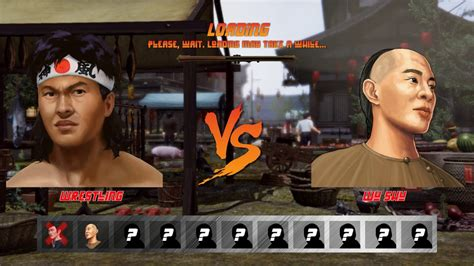### Shaolin Long Fist

Shaolin Long Fist
QCF+W
D*W (LOW)
U+W,D+W
B)+WW Pause till second hit lands>B+W
B+W on second hit B+W alternate combo ender for the last hit of a kick or punch combo
WWW >W(OVERHEAD) >D+W >U+W,D+W >B+W on second hit B+W >F)+WWW or B+W on second hit B+W or >U+W,D+W
U+W,D+W
B+W on second hit B+W
QCB+P
QCF+P ( first hit unblockable) *Probably ment to be this way
PPP >D)+PPPPPP U)+PPP D+K,F)+KK or B+K or ~U+K
F)+PPP >P >U)+PPP >B)+PPP >D)+PPPPPP OR B+PPP OR U+PPP ~PPP
D*P(LOW) PPPP,P or K or B)+PPP or U)+PPP or B+K or F)+KK orB)+WWpause till 2nd hitB+W
QCB+K
QCF+K
KK >K >P >U)+PPP >B)+PPP >B+W on second hit B+W
KK,F+K >K B+K >U+K >F+K >P >U)+PPP >B+W on second hit B+W
F+K F+K >K B+K >U+K >F+K >P >U)+PPP >B+W on second hit B+W
K*K B+K F)+KK B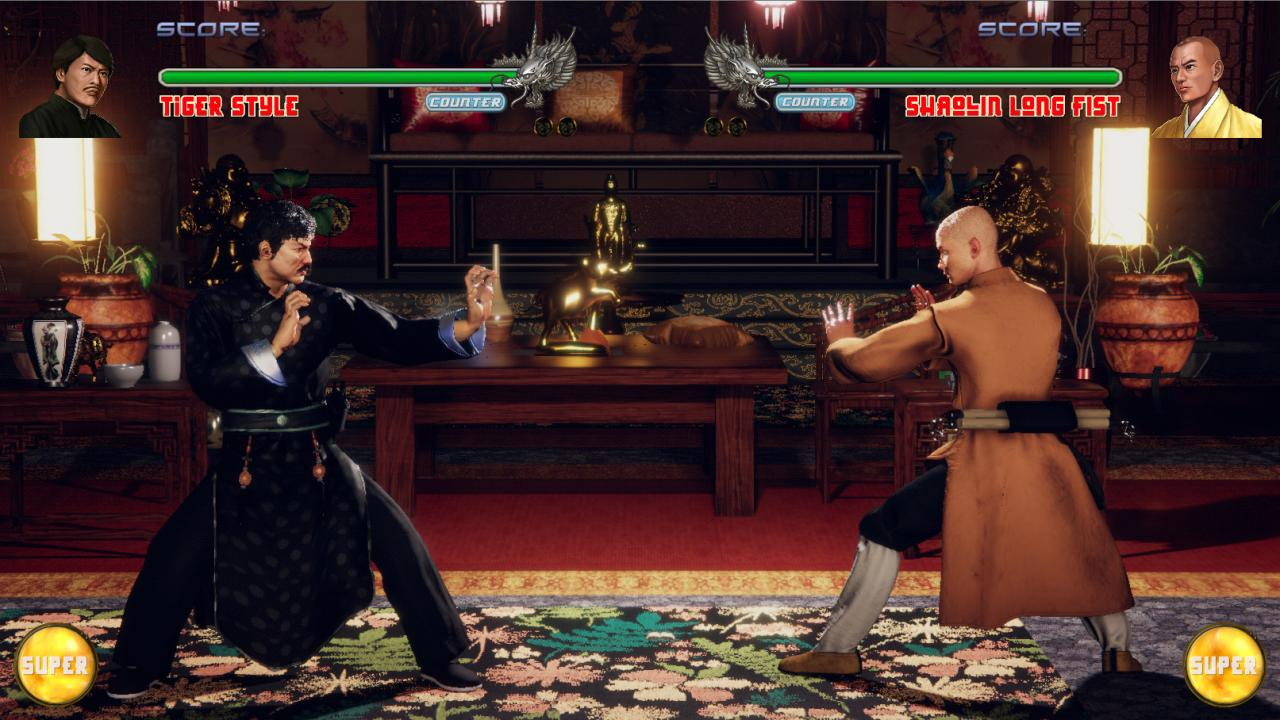### Wu Shu

Wu Shu
ACF+W
D*W >D)+WW >K
U+W (overhead) >U+W >K >D)+WWW B)+WWW~(last hit does not combo)
K Combo ender for weapon attacks
WW >D)+WW B)+WWW (4th hit no combo) >F)+WWW >F)+WW,D)+WW OR B)+WW >U)+WW
WWW >D)+WW
B)+WWW~ alternate combo ender for the last hit of a kick or punch combo
QCB+P
QCF+P >P
D*P Doesn’t apear to combo into anything or combo into by pressing D+P
B+P alternate combo ender for the last hit of a kick or punch combo
PPP U+P >K or >B+K~K
PPPP >~P >KKKK,~K or D)+KK >F)+PPPP,~P or B+P >KK,D+K >U)+K~K U+P >K or >B+K~K
F)+PPPP >F+P >P >B+P >KKK
U+P >K or >B+K~K
ACB+K >K
QCF+K >K
KK >U+P,K or B+K
KKKK ~K >D)+KK
F)+K >KK,K or U+K~U+K >U+K~U+K
F)+KKK(LOW)K >K >P D)+KK >U+K~U+K >B+K~B+K
U+K ~U+K >B+P >B+WWW
B)+K~K
D*K(LOW) >K >B)+WW
D*P Doesn’t apear to combo into anything or combo into by pressing D+P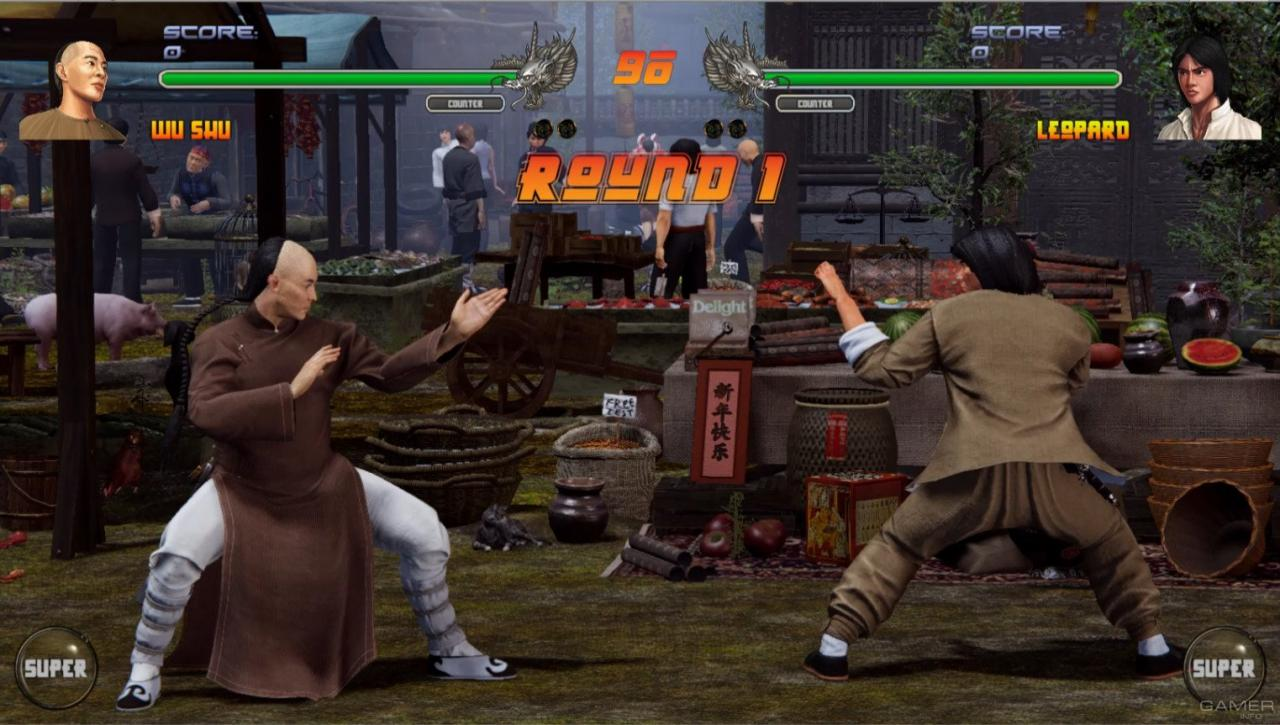### Dang Soo Do

Dang Soo Do
U+W
D*W
W >B+W
WW >W >B+W >F)+WW,D+W or B+W ~U+W
QCF+P
PP >U)+PPPP >D+K,D)+K~K or B+P or B+W or ~B+K or ~U+K
PPP ~P >KKK~K >D+KKK >D+P,K >B+W >D+K,D)+K~K or B+P or B+W or ~B+K or ~U+K
F+P,D+P,K
F)+PP >U)+PPPP >D+P,K
F)+PPP >KK >B+K >U+K >B+W >D+P,K
F)+PPPP >P >K,P >B+K >B+P
D*P >K >B+W really close
QCB+K
QCF+K (Second hit misses on crouching opponent)
KKK >P ~K ~F+K ~B+K
F)+KK >B+P >B+K
F)+KKK >F+K >B+K ~U+K ~KK >PP,B+P or B+K
D*K >KK >B+P >B+W ~B+K ~U+K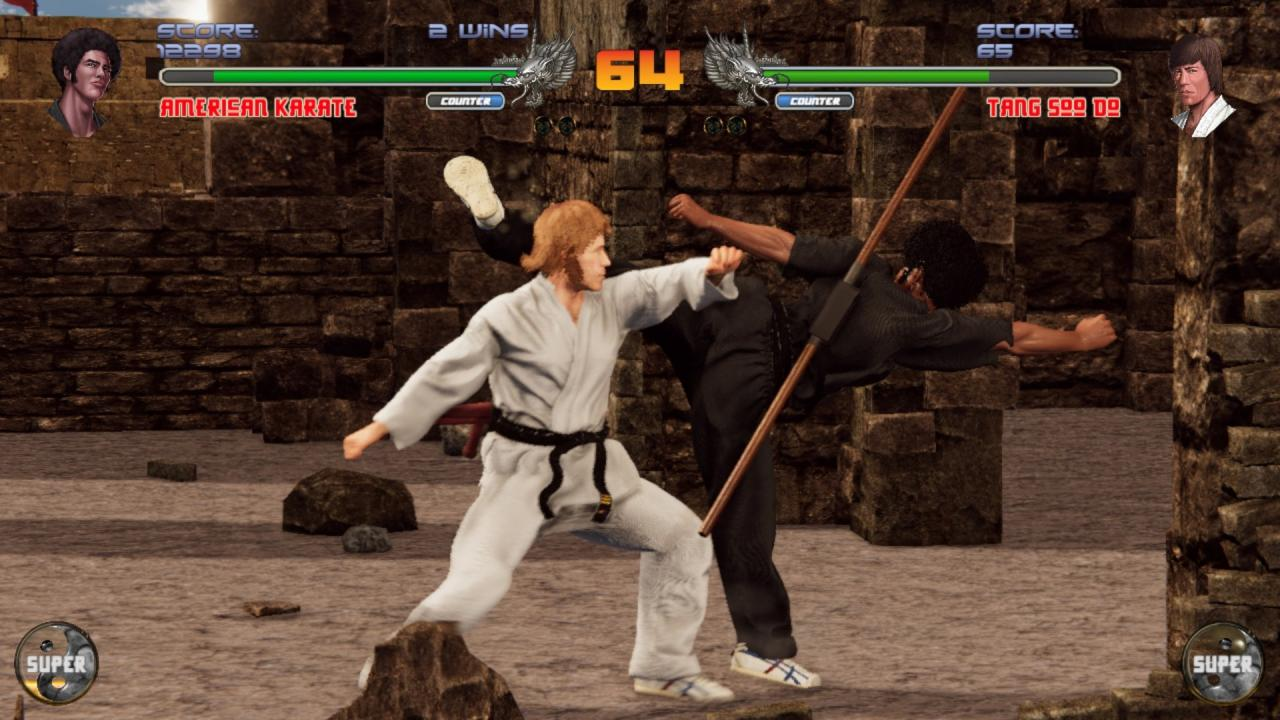### Lizard Style

Lizard Style
D*W
WW >B+W >U+W ~D+W
F+W > >U+W >B+W
F)+WW >W >B+W
QCB+P >P
QCF+P
D*P ~K
U+P (slight pause till head lifts up) then >B+PPP >U+K ~U+P
D*P(LOW) ~K >B)+PPP
QCB+K
QCF+K
F+K >KK
KK >D)+KK >U)+KK ~B+W >P,~P or B)+PPP
KKK U+K >U+P,U_K ~KK B)+KK
D*K(LOW) >K
F+K >F)+KK >K~K >P,B)+PPP >P,U+K
Note: Third and forth hit of super hit low.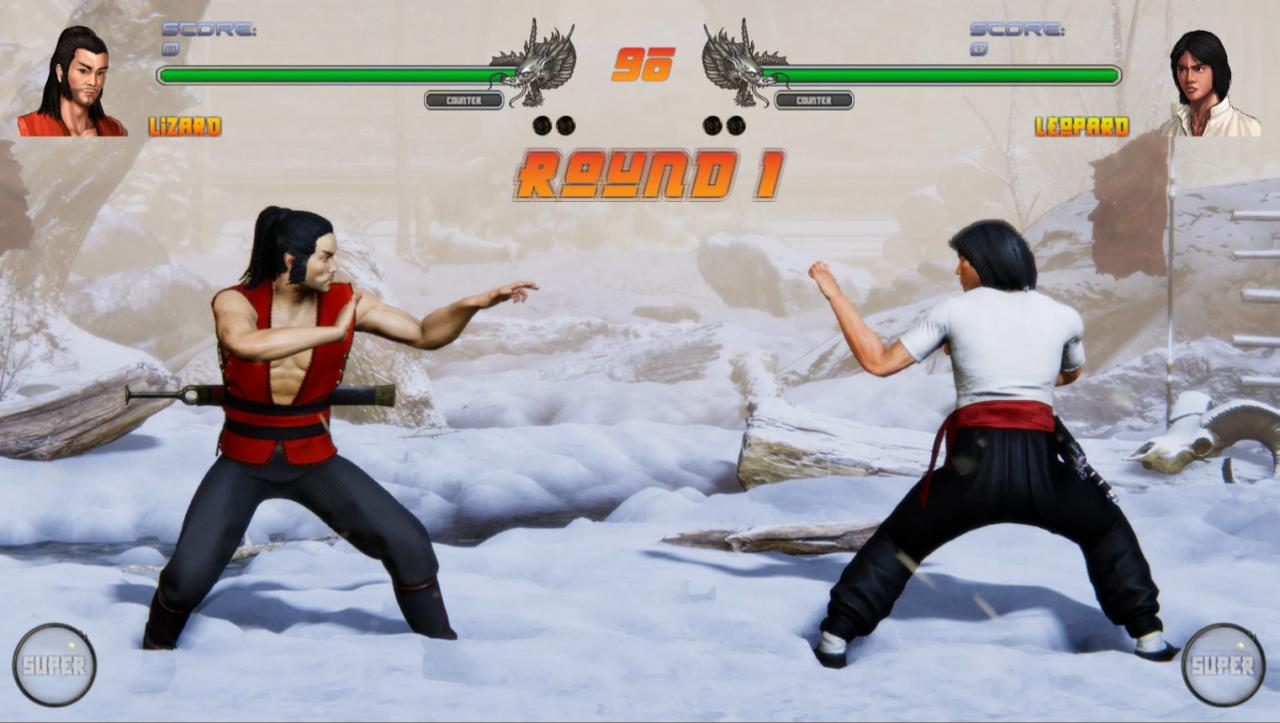### Monkey Style

Monkey Style
Dash >K,K
>D+W >B+W F+W,D+W OR U+W OR B+W
B+W
QCB+K(character bounces of the cround and you can hit with a low hiting move) >QCF+K
QCF+K
KK >D+K,K
F)+KK(LOW) ~F+K(second hit is an overhead) >KK U+K >D)+KK B)+KK
D*K(LOW) >K >U+K
D*P(LOW) >PP(low) >B)+PP >P,D+K,K ~U+W
QCF+P
F)+PP >KKKK
F)+PPP ~F+P >P,P OR D+K,K or ~K >U)+PP B)+PP
Note: Second and Third hit of super hit low.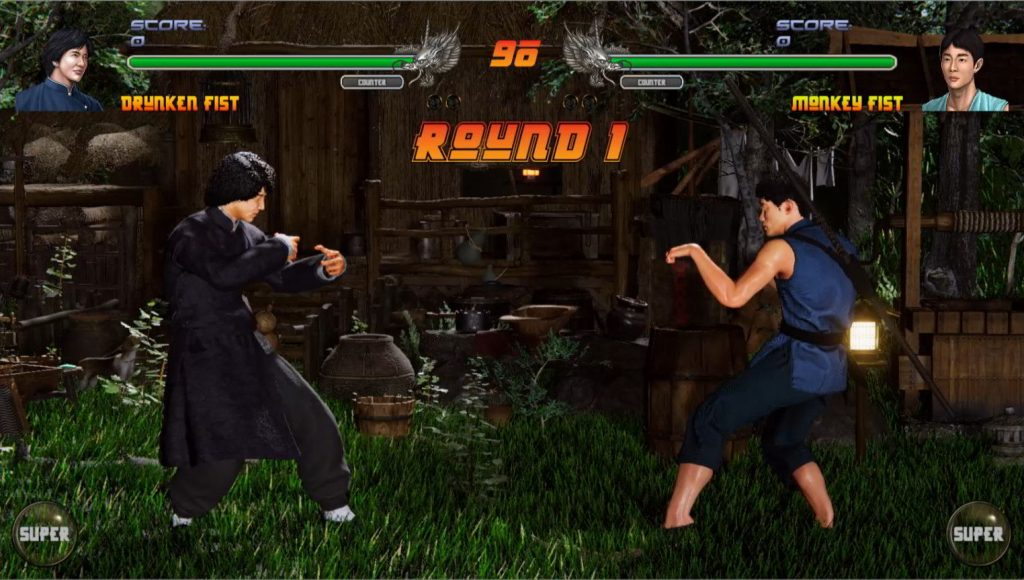### Tiger Style

Tiger Style
QCF+W
F+WW
D*W
WW >WW ~>B+W ~U+W ~D+W
QCB+P
QCF+P
PPP >P B)+PP D)+PP ~U+P >KKK
F)+PP >D)+PP
F)+PPP ~PP >F)+KK
F)+PPPP(First hit overhead) >K >U+K >B+K ~PP
B+P >P >P >K >B+W >U+W
D*P(LOW) >P >B+PP >B+W >U+W >U+K >B+K >F)+KK
QCB+K
QCF+K
B+K
U+K
KK >K >D+K,F)+KK or U+K or B+K >D+K,U+K or B+K
F)+KK >K >P >B+PP >B+W >U+W >U+K >B+K
D*K(NOT LOW) >P >B+PP >B+W >U+W >U+K >B+K >F)+KK or U+K or B+K
D+K Combo linker for punch and kicks
Note: Third and fouth hit of super hit low.### Drunken Fist

Drunken Fist
Dash > P >K
Dash, Dash(IT HITS TWICE) >KKKK,U+KD+K or B+K or F)+KKK >F)+PP,D+P
QCB+W
QCF+W
D*W
WW F)+WW,~W or U+P or D+P
F+W >U+W >B+W
F)+WW( have to be very close to connect second hit) ~P(overhead) >U+P >D+P
QCB+P
QCF+P
U+K(overhead) >B+K >D+K >B+K ~*P as rising
PPP >P U+P,PPP >B)+WW >K,U+K >KKKK,U+KD+K or B+K or F)+KKK or B+K
F)+PP >P ~F)+PP(overhead) U+P,PPP D)+PPP or K >B)+WW
B+P >P >B+P >B+K
U+P >PPP >KKK ~B+P ~D+
D*P >P >K
QCB+K
QCF+K
U+K
B+K
Back Dash,Back Dash >P
KKK >D+K >P B)+PP
KKKK >U+KD+K >B+K >F)+KKK or B+K
F+K ~P
F)+KK >/~P >F+K >B+K >U+K >KK(overhead) or B+K
D*K >K D+K B)+PP
NOTES:
First swing up of super doesn’t hit.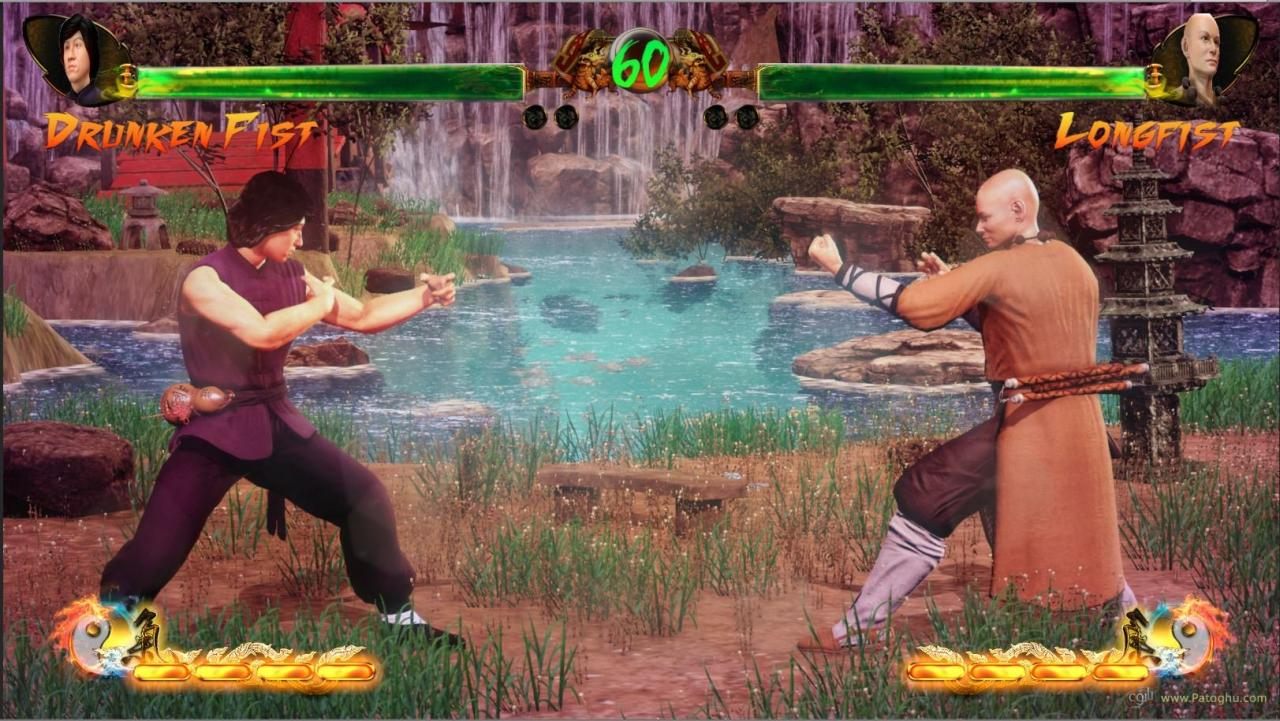### Muay Thai

Muay Thai
QCB+W
QCF+W
B+W
D*W(LOW)(Very close)>B+W ~U+W
W (Pause) B+W >F+WW
WW >W ( For the rest to come out you need to pause after second hit) >B+W ~U+W ~D+W ~F+WW
QCB+P
QCF+P
D*P
PP >B+P >U+P >D+P
PPP >P(overhead) >D+P >U+P >B+P >KKKK
F)+PPP >P(overhead) >P,P or U+P >K
QCB+K
QCF+K
D)*KK,D+K(LOW)or F+P or B+P or B+W
D*K >U+K >U+P >B+P >F+P
KKK >K >U+K,B+K or B+W >D)+KKK >D+P >U+P >B+P >B+W
KKKK (very quickly press)->D)+KKK >U+K
F+K >K >PP >B+P >U+P >B+W
F)+KK ~F+K >PP >D)+KKK >B+P ~U+P ~B+W### Ninjutsu

Ninjutsu
Dash >P Hits low
Dash near >P in the air
backdash,backdash >K in the air
QCB+W
QCF+W
WW >W U+W D+W ~B+W
QCB+P
QCF+P (appears unblockable)*maybe because its a throwing star
D*P
PPP >P >D+KK >B)+PP >B+WK,P or ~K
F)+PP >P >K >F)+KK,U+K or D+K or ~K >KKKK
B+P >P >/~B+W >K,P or ~K